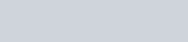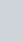A number is selected from numbers 1 to 25. The probability that it is prime is
Question:

A number is selected from numbers 1 to 25. The probability that it is prime is

(a) $\frac{2}{3}$

(b) $\frac{1}{6}$

(c) $\frac{1}{3}$

(d) $\frac{5}{6}$

Solution:

GIVEN: A number is selected from 1 to 25

TO FIND: Probability that, the number is a prime

Total number is 25

Numbers from 1 to 25 that are primes are 2, 3, 5, 7, 11, 13, 17, 19 and 23

Total numbers that are primes from 1 to 25 is 9

We know that PROBABILITY =Hence probability of getting a prime number from 1 to 25 is equal toHence no option is correct